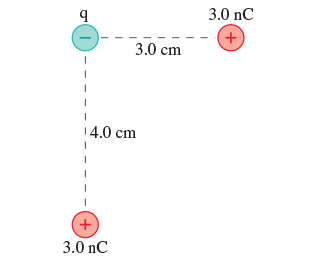# Problem: What is the electric potential energy of the group of charges in (Figure 1) ? Assume that q = -5.5 nC.

###### FREE Expert Solution

This problem involves electric potential energy. The approach is simple and straight forward.

Electric potential energy:

81% (87 ratings)###### Problem Details

What is the electric potential energy of the group of charges in (Figure 1) ? Assume that q = -5.5 nC.